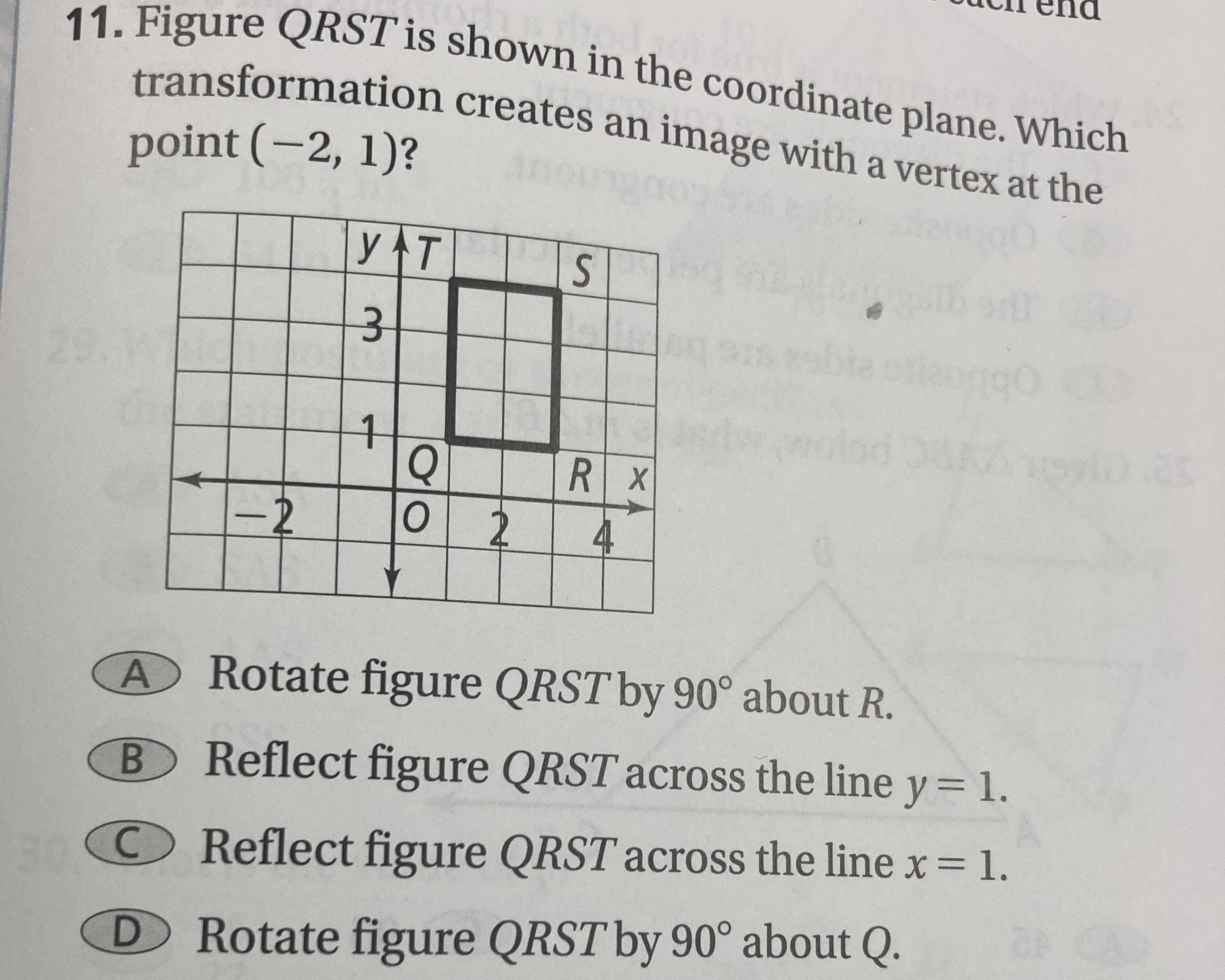### ¿Todavía tienes preguntas de matemáticas?

Pregunte a nuestros tutores expertos
Algebra
Pregunta1. Figure $$Q R S T$$ is shown in the coordinate plane. Which transformation creates an image with a vertex at the point $$( - 2,1 )$$ ?

(A) Rotate figure $$Q R S T$$ by $$90 ^ { \circ }$$ about $$R$$ .

(B) Reflect figure $$Q R S T$$ across the line $$y = 1$$ .

(C) Reflect figure $$Q R S T$$ across the line $$x = 1$$ .

(D) Rotate figure $$Q R S T$$ by $$90 ^ { \circ }$$ about $$Q$$ .